Yarn Numbering System | Textile Study Center
Home Fabric Engineering Yarn Numbering System# Yarn Numbering System

39.34K
0#### Md Sohanur Rahman Sobuj

Co-founder , Admin & Author at Textile Study Center
Student of Bangladesh University of Textiles (BUTEX) Department: Apparel Engineering#### Latest posts by Md Sohanur Rahman Sobuj (see all)

Yarn Number

Yarn number is a measure of the fineness or size of a yarn expressed either as mass per unit length or length per unit. Yarn Count and Yarn Size are synonymous with Yarn Number.

Yarn Numbering System

There are two systems of expressing yarn number or yarn count.

Direct yarn numbering system (mass/unit length)

Indirect yarn numbering system (length/unit mass)

Direct yarn numbering system

In a direct yarn counting system, the yarn number or count is the weight of a unit length of yarn. This means the higher the yarn count number, the heavier or thicker the yarn. It is fixed length system. This system is generally used for jute or silk yarn.

The following formula is used to calculate direct yarn count system.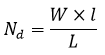Where, w=yarn number or count

W=the weight of the sample (yarn) in units of the system at the official regain

L=length of the sample, and

l=unit of length of the system

Major direct numbering System

Denier: In the direct Denier system, the yarn count number indicates “the weight in grams of 9000 meters of yarn”.

e.g. 30D indicates that 9000 meters of yarn weight 30 grams.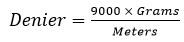Tex: In the direct universal Tex system, the yarn count number indicates “the weight in grams of 1000 meters of yarn”.

e.g. 30 Tex indicates that 1000 meters of yarn weight 30 grams.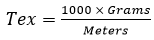Dtex: In the direct Decitex system, the yarn count number indicates “the Adobe Illustrator weight in grams of 10000 meters of yarn”. (1 dtex=0.9 denier)

e.g. 50 Tex indicates that 1000 meters of yarn weight 30 grams.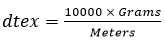SL Name of the system Unit of the system ( W ) Unit of Length ( l ) 1 ( Denier D or Td ) No. of grams 9000 m 2 Tex No. of grams 1000 m 3 dtex No. of grams 10000 m 4 Millitex ( mtex ) No. of milligrams 1000 m 5 Kilotex ( ktex ) No. of kilograms 1000 m 6 Grist ( for jute yarn ) No. of pounds ( lbs ) 14400 yds 7 Silk Count No of drams ( =1/16 ounce ) 1000 yds

Math:  If a skein of 100 m of filament Viscose Yarn weight is 1.67 g, calculate its Denier.

SolnIn the Denier system the weight unit is the gram and the unit of length is 9000 m.

Thus, W=1.67 g, L=100 m, and  = 9000 m.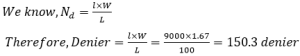So the yarn count is 150.3 D.

Indirect yarn numbering or counting system

In an indirect yarn counting system, the yarn number or count is the number of “units of length” per “unit of weight” of yarn. This means the higher the yarn count number, the finer or thinner the yarn. It is based on the fixed weight system. This system is generally used for cotton, woolen, worsted and linen yarn.

The following formula is used to calculate indirect yarn count system.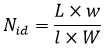Where, Nid=yarn number or count in indirect system

W=the weight of the sample (yarn) in units of the system at the official regain

w=unit of weight of the system

L=length of the sample, and

l=unit of length of the system

Major indirect numbering or counting System

English Cotton Count (Ne): In the indirect English cotton count system, the yarn count number indicates “number of 840 yard hanks of yarn per 1 pound weight”.

e.g. 30/1 cotton(1 means single yarn) indicates that 30 x 840 yards of yarn weight 1 pound.

e.g. 40/2’s (2 means ply yarn) indicates that 20(Resultant count)x 840 yards of yarn weight 1 pound.Metric Count (Ne): In the indirect metric count system, the yarn count number indicates “number of 1000 m (or 1 Km) of yarn per 1 Kg weight”.

e.g. 30 Nm indicates that 30 kilometers or 30000 meters of yarn weight 1 kilogram.For example, if we consider a yarn of 2000m having the weight of 450g. So the count in metric system will beWorsted Count: In the indirect worsted count system, the yarn count number indicates “number of 560 yards hanks of yarn per 1 pound weight”.

e.g. 1/20 worsted indicates that 20 x 560 yards of yarn weight 1 pound.

e.g. 3/40 worsted indicates that 13.33(Resultant count)x560 yards of yarn weight 1 pound.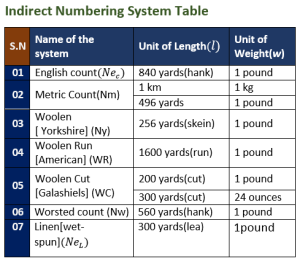e.g. 50 Nm Metric indicates that 50 x 256 yards of yarn weight 1 pound.

e.g. 15 cut Galashiels indicates that 15 x 200 yards of yarn weight 1 pound.

e.g. 16 skeins Yorkshire indicates that 16 x 256 yards of yarn weight 1 pound.

e.g. 10 lea Linen indicates that 10 x 300 yards of yarn weight 1 pound.

Note: Woolen and Worsted Systems-The basic difference between the two is that in the Worsted system all short fibers are removed and the remaining long ones are aligned parallel. In the Woolen system there is no removal of short fibers, so some fibers lie parallel and others randomly.

Math: A lea (120 yd) of cotton yarn weighs 25 gr, calculate its count in the cotton system.

Soln: In English cotton count system, the ‘unit of length’ is the hank (840 yd) and the ‘unit of weight’ is 1 lb. In 1 lb there are 7,000 grains.

Hence, L =120 yd, =840 yd, W=25/7,000 lb, and w=1 lb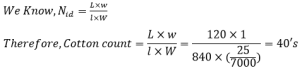So the yarn count is 40’s.

(39339)#### Md Sohanur Rahman Sobuj

Co-founder , Admin & Author at Textile Study Center
Student of Bangladesh University of Textiles (BUTEX) Department: Apparel Engineering#### Latest posts by Md Sohanur Rahman Sobuj (see all)Close
Share via